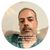# App Development

cancel
Showing results for
Did you mean:Creator

## Block 0 value lines?In above chart, I want to remove lines where there is no delta in qty.

Detla qty is    =sum(OurReqQty)-sum(Open_Order_Quantity__c)

I have tried this

if( sum({1}OurReqQty)-Sum({1}Open_Order_Quantity__c)=0,0,sum(OurReqQty)-Sum(Open_Order_Quantity__c))

and switched off 'show zero values' in Add-ons, but didn't work. A similiar approach worked on another app recently, but not this time.

Any help appreciated. thanks.

4 RepliesMVP

Do you have any other measure with non zero values, I think it only switches off zero values when all measures in a row produces 0 values.Contributor III

Stephen,

You will need to wrap all the expressions in this chart in If statements like this:

if( sum(OurReqQty)-Sum(Open_Order_Quantity__c)=0,0,sum(OurReqQty)-Sum(Open_Order_Quantity__c))

if( sum(OurReqQty)-Sum(Open_Order_Quantity__c)=0,0,sum(EpicorValue))

I don't think the set analysis expression is needed in the If condition - if( sum( {1} ...

Without it, the If condition will use the same set of data that the rest of the expression does, so each row shows or hides as it should.

I hope that helps.Creator
Author

Thanks for you reply, now resolved, as I removed the other columns.Creator
Author

Thanks for you reply, this worked great